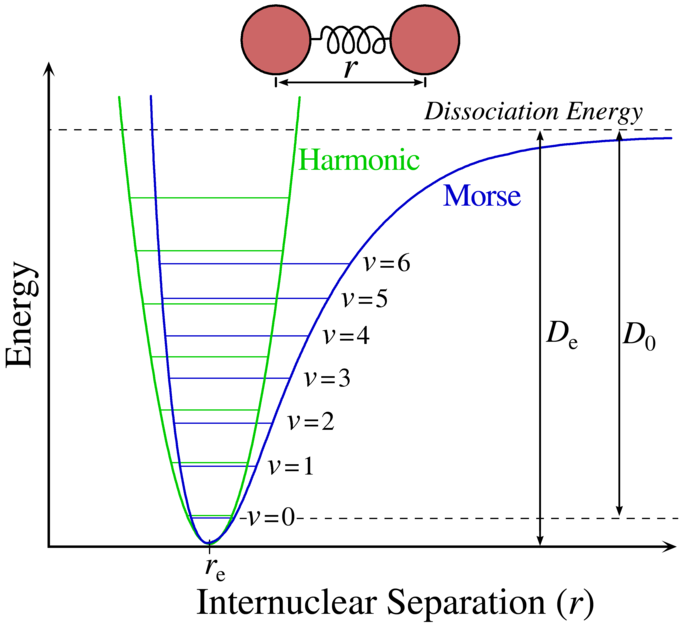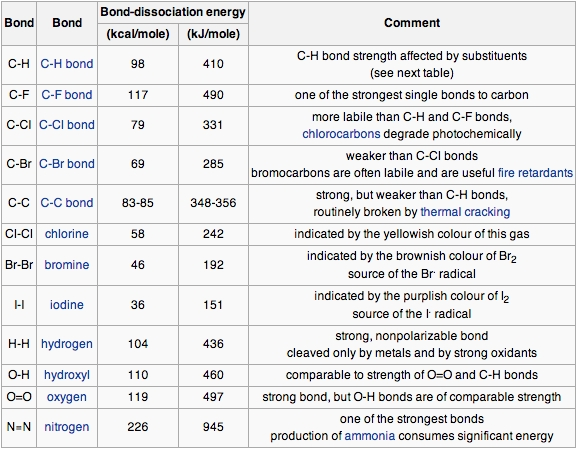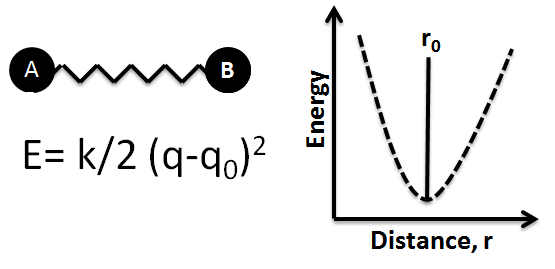## Bond Energy

Bond energy is the measure of bond strength. In order to turn one mole of a molecule into its constituent atoms, an amount of heat equal to the bond energy needs to be put into the system.

### Learning Objectives

Identify the relationship between bond energy and strength of chemical bonds

### Key Takeaways

#### Key Points

• Values listed in tables of bond energy and bond length are averages taken over a variety of compounds that contain a specific atom pair.
• A plot of the potential energy of a two-atom system and the distance between the atoms reveals a distance at which the energy is at its minimum. This distance is the bond length between the atoms.
• The higher the bond energy associated with a specific atom pair, the stronger the bond is said to be, and the smaller the distance between the two atoms.

#### Key Terms

• equilibrium bond length: The average distance between two atoms when they are bonded to each other.
• Morse curve: A plot showing the dependence of the energy associated with a system of two atoms on the distance between them (referred to as the ‘internuclear distance’).
• enthalpy: In thermodynamics, a measure of the heat content of a chemical or physical system, measured under conditions of constant pressure.
• Bond Energy: A measure of a chemical bond’s strength. It is experimentally determined by measuring the heat (or enthalpy) required to break a mole of molecules into their constituent individual atoms.

### The Energy Associated with a Chemical Bond

Bond energy is a measure of a chemical bond ‘s strength, meaning that it tells us how likely a pair of atoms is to remain bonded in the presence of energy perturbations. Alternatively, it can be thought of as a measure of the stability gained when two atoms bond to each other, as opposed to their free or unbound states.

Bond energy is determined by measuring the heat required to break one mole of molecules into their individual atoms, and it represents the average energy associated with breaking the individual bonds of a molecule. The higher the bond energy, the ‘stronger’ we say the bond is between the two atoms, and the distance between them (bond length) is smaller.

For instance, the HO-H bond in a water molecule requires 493 kJ/mol to break and generate the hydroxide ion (OH). Breaking the O-H bond in the hydroxide ion requires an additional 424 kJ/mol. Therefore, the bond energy of the covalent O-H bonds in water is reported to be the average of the two values, or 458.9 kJ/mol. These energy values (493 and 424 kJ/mol) required to break successive O-H bonds in the water molecule are called ‘ bond dissociation energies,’ and they are different from the bond energy. The bond energy is the average of the bond dissociation energies in a molecule.

The exact properties of a specific kind of bond are determined in part by the nature of the other bonds in the molecule; for example, the energy and length of the C–H bond will vary depending on what other atoms are bonded to the carbon atom. Similarly, the C-H bond length can vary by as much as 4% between different molecules. For this reason, the values listed in tables of bond energy and bond length are usually averages taken over a variety of compounds that contain a specific atom pair.

We can apply bond energy values to determine the enthalpy of a compound’s formation, $\Delta H_f$, which can be roughly approximated by simply adding tabulated values for the bond energies of all created bonds. The accuracy of this method is within a few percent of the experimentally determined $\Delta H_f$ values.

### Energy Between Two Atoms as a Function of Internuclear Distance

A Morse curve shows how the energy of a two atom system changes as a function of internuclear distance.Morse curve: Plot of potential energy vs distance between two atoms. The bond energy is energy that must be added from the minimum of the ‘potential energy well’ to the point of zero energy, which represents the two atoms being infinitely far apart, or, practically speaking, not bonded to each other.

At large distances, the energy is zero, meaning that there is no interaction. This agrees with our understanding that two atoms placed infinitely far apart do not interact with each other in any meaningful way, or at the very least we can say that they are not bonded to each other. At internuclear distances in the order of an atomic diameter, attractive forces dominate. At very small distances between the two atoms, the force is repulsive and the energy of the two atom system is very high. The attractive and repulsive forces are balanced at the minimum point in the plot of a Morse curve.

The internuclear distance at which the energy minimum occurs defines the equilibrium bond length. This bond length represents an ‘equilibrium’ value because thermal motion causes the two atoms to vibrate about this distance, much like a spring vibrates back and forth around its unstretched, or equilibrium distance.

A Morse curve will have different energy minima and distance dependence for bonds formed between different pairs of atoms. In general, the stronger the bond between two atoms, the lower the energy minimum is and the smaller the bond length.

The bond energy is the amount of work that must be done to pull two atoms completely apart; in other words, it is the same as the depth of the “well” in the potential energy curve.

## Bond Enthalpy

Bond enthalpy is defined as the enthalpy change when a covalent bond is cleaved by homolysis.

### Learning Objectives

Describe the changes in enthalpy accompanying the breaking or formation of a bond

### Key Takeaways

#### Key Points

• Breaking a bond requires the input of energy (positive change in enthalpy ); energy is released (negative change in enthalpy) when forming a bond.
• Bond enthalpy, or dissociation energy, is defined as the standard enthalpy change when a bond is cleaved by homolysis, with reactants and products of the homolysis reaction at 0 K (absolute zero).

#### Key Terms

• enthalpy: A measure of the heat energy content in a thermodynamic system.
• homolysis: The symmetrical breaking of a chemical bond within a molecule so that each of the resulting fragments retains one of the originally bonded electrons.
• exothermic: A chemical reaction that releases heat energy to its surroundings.
• endothermic: A chemical reaction that absorbs heat energy from its surroundings.

### Change in Enthalpy

Enthalpy is a measure of the total heat energy content in a thermodynamic system, and it is practically used to describe energy transfer during chemical or physical processes in which the pressure remains constant.

The total enthalpy, H, of a system cannot be measured directly. Thus, the change in enthalpy, $\Delta H$, is a more useful quantity than its absolute value. The change ($\Delta H$) is positive in endothermic reactions because the products of the reaction have a greater enthalpy than the reactants, and heat is absorbed by the system from its surroundings. The change in enthalpy is negative in exothermic processes, because energy is released from the system into its surroundings.

Generally, a positive change in enthalpy is required to break a bond, while a negative change in enthalpy is accompanied by the formation of a bond. In other words, breaking a bond is an endothermic process, while the formation of bonds is exothermic.

### Bond Enthalpy or Dissociation Energy

Bond enthalpy, also known as bond dissociation energy, is defined as the standard enthalpy change when a bond is cleaved by homolysis, with reactants and products of the homolysis reaction at 0 K (absolute zero).Homolysis of a chemical bond: A two-electron covalent bond is equally split when bond breaking, with each resulting fragment having one electron from the original shared pair. Notice that the products are free-radicals.

For instance, the bond enthalpy, or bond-dissociation energy, for one of the C-H bonds in ethane (C2H6) is defined by the process:

$\text{CH}_3\text{CH}_2-\text{H} \rightarrow \text{CH}_3\text{CH}_2 \cdot + \text{H}$

$\Delta H = 101.1 \frac{\text{kcal}}{\text{mol}} \left(423.0 \frac{\text{kJ}}{\text{mol}}\right)$

The strength of bonds between different atoms varies across the periodic table and is well documented.Bond dissociation energy: Bond dissociation energies for different element pairings are listed. It is evident that bond strength varies significantly for different combinations of elements in the periodic table.

Each bond in a molecule has its own bond dissociation energy, so a molecule with four bonds will require more energy to break the bonds than a molecule with one bond. As each successive bond is broken, the bond dissociation energy required for the other bonds changes slightly.

## Bond Lengths

Bond length between two atoms depends on factors such as the orbital hybridization and the electronic nature of the components.

### Learning Objectives

Compare bond lengths using knowledge of periodic trends

### Key Takeaways

#### Key Points

• Bond length is the experimentally determined average distance between two bonded atoms.
• Bonded atoms vibrate due to thermal energy available in the surroundings.
• Bond lengths are typically in the range of 100-200 pm (1-2 Å).
• As a general trend, bond length decreases across a row in the periodic table and increases down a group.
• Atoms with multiple bonds between them have shorter bond lengths than singly bonded ones.

#### Key Terms

• bond length: In molecular geometry, bond length or bond distance is the average distance between nuclei of two bonded atoms in a molecule.
• covalent radius: The radius of an atom when covalently bonded to other atoms.

### The Distance Between Two Bonded Atoms

The distance between two atoms participating in a bond, known as the bond length, can be determined experimentally. X-ray diffraction of molecular crystals allows for the determination of the three-dimensional structure of molecules and the precise measurement of internuclear distances. Various spectroscopic methods also exist for estimating the bond length between two atoms in a molecule.

### Bonds Are Not Static Structures

The bond length is the average distance between the nuclei of two bonded atoms in a molecule. This is because a chemical bond is not a static structure, but the two atoms actually vibrate due to thermal energy available in the surroundings at any non-zero Kelvin temperature. A bond can be modeled as two balls connected by a spring: stretching or compressing the spring initiates a back-and-forth motion with respect to the equilibrium positions of the balls. Measured bond lengths are the distance between those unperturbed, or equilibrium, positions of the balls, or atoms.Ball-and-spring model of a chemical bond: A bond between two atoms can be thought of as a spring with two balls attached to it. Any stretch or compression of the spring will initiate oscillations of the atoms with respect to their equilibrium (unperturbed) positions. The potential energy function for this system is also indicated. The minimum energy occurs at the equilibrium distance r0, which is where the bond length is measured.

### Bond Length Values

Bond lengths have traditionally been expressed in Ångstrom units, but picometers are sometimes preferred (1 Å = 10-10 m = 100 pm). Bonds lengths are typically in the range of 1-2 Å, or 100-200 pm. Even though the bond vibrates, equilibrium bond lengths can be determined experimentally to within ±1 pm. The actual distance between two atoms in a molecule depends on factors such as the orbital hybridization and the electronic nature of its components.

Bonds involving hydrogen can be quite short; the shortest bond of all, H–H, is only 74 pm. The covalent radius of an atom is determined by halving the bond distance between two identical atoms. Based on data for the H2 molecule, the covalent radius of H is 37 pm. Covalent radii can be used to estimate the bond distance between two different atoms; it is the sum of the individual covalent radii.

### Periodic Trends

Generally, when we consider a bond between a given atom and a varying atomic bonding partner, the bond length decreases across a period in the periodic table, and increases down a group. This trend is identical to that of the atomic radius.

Atoms with multiple bonds between them have shorter bond lengths than singly bonded ones; this is a major criterion for experimentally determining the multiplicity of a bond. For example, the bond length of $\text{C}-\text{C}$ is 154 pm; the bond length of $\text{C}=\text{C}$ is 133 pm; and finally, the bond length of $\text{C}\equiv\text{C}$ is 120 pm.